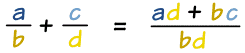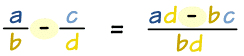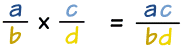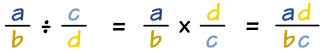# Fractions in Algebra

We can add, subtract, multiply and divide fractions in algebra in the same way we do in simple arithmetic.

## Adding Fractions

To add fractions there is a simple rule:(See why this works on the Common Denominator page).

### Example:

x 2 + y 5 = (x)(5) + (2)(y) (2)(5)

= 5x+2y 10

### Example:

x + 4 3 + x − 3 4 = (x+4)(4) + (3)(x−3) (3)(4)

= 4x+16 + 3x−9 12

= 7x+7 12

## Subtracting Fractions

Subtracting fractions is very similar, except that the + is now −### Example:

x + 2 x  −  x x − 2  =  (x+2)(x−2) − (x)(x) x(x−2)

(x2 − 22) − x2 x2 − 2x

−4 x2 − 2x

## Multiplying Fractions

Multiplying fractions is the easiest one of all, just multiply the tops together, and the bottoms together:### Example:

3x x−2  ×  x 3  =  (3x)(x) 3(x−2)

3x2 3(x−2)

x2 x−2

## Dividing Fractions

To divide fractions, first "flip" the fraction we want to divide by, then use the same method as for multiplying:### Example:

3y2 x+1  ÷  y 2  =  3y2 x+1  ×  2 y

= (3y2)(2) (x+1)(y)

= 6y2 (x+1)(y)

6y x+1

Hard: